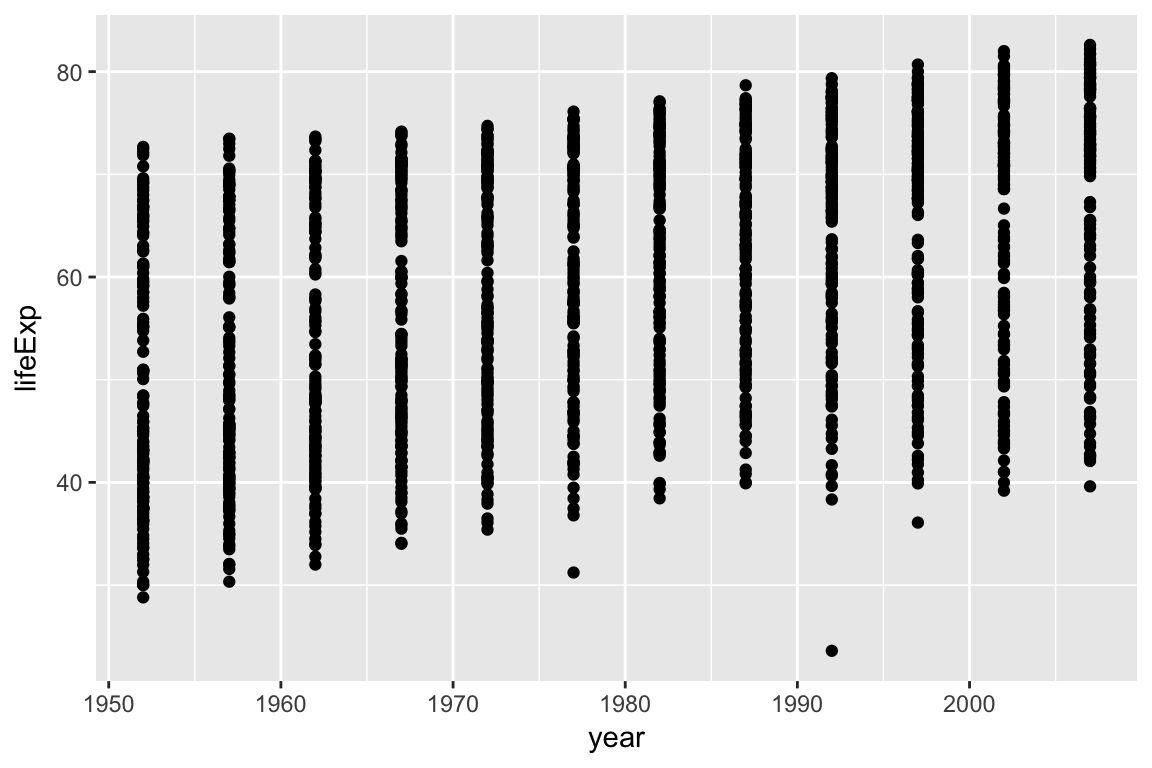We already saw some of R’s built in plotting facilities with the function `plot`. A more recent and much more powerful plotting library is `ggplot2`. `ggplot2` is another mini-language within R, a language for creating plots. It implements ideas from a book called [“The Grammar of Graphics” [url https://www.amazon.com/Grammar-Graphics-Statistics-Computing/dp/0387245448]]. The syntax can be a little strange, but there are plenty of examples in the online documentation.

If `ggplot2` isn’t already installed, we would need to install it.

``````# install.packages("ggplot2")
# or
# install.packages("tidyverse")``````

`ggplot2` is part of the Tidyverse, so loadinging the `tidyverse` package will load `ggplot2`.

``library(tidyverse)``
``## ── Attaching packages ────────────────────────────────── tidyverse 1.2.1 ──``
``````## ✔ ggplot2 2.2.1     ✔ purrr   0.2.4
## ✔ tibble  1.3.4     ✔ dplyr   0.7.4
## ✔ tidyr   0.7.2     ✔ stringr 1.2.0
## ✔ readr   1.1.1     ✔ forcats 0.2.0``````
``````## ── Conflicts ───────────────────────────────────── tidyverse_conflicts() ──

Producing a plot with `ggplot2`, we must give three things:

1. A data frame containing our data.
2. How the columns of the data frame can be translated into positions, colors, sizes, and shapes of graphical elements (“aesthetics”).
3. The actual graphical elements to display (“geometric objects”).

# Plotting with ggplot2

We continue using the Gapminder data, which was loaded with:

``gap <- read_csv("gapminder.csv")``
``````## Parsed with column specification:
## cols(
##   country = col_character(),
##   continent = col_character(),
##   year = col_integer(),
##   lifeExp = col_double(),
##   pop = col_integer(),
##   gdpPercap = col_double()
## )``````

Let’s make our first ggplot.

``````ggplot(gap, aes(x=year, y=lifeExp)) +
geom_point()``````The call to `ggplot` and `aes` sets up the basics of how we are going to represent the various columns of the data frame. `aes` defines the “aesthetics”, which is how columns of the data frame map to graphical attributes such as x and y position, color, size, etc. We then literally add layers of graphics to this.

`aes` is another example of magic “non-standard evaluation”, arguments to `aes` may refer to columns of the data frame directly.

Further aesthetics can be used. Any aesthetic can be either numeric or categorical, an appropriate scale will be used.

``````ggplot(gap, aes(x=year, y=lifeExp, color=continent, size=pop)) +
geom_point()``````